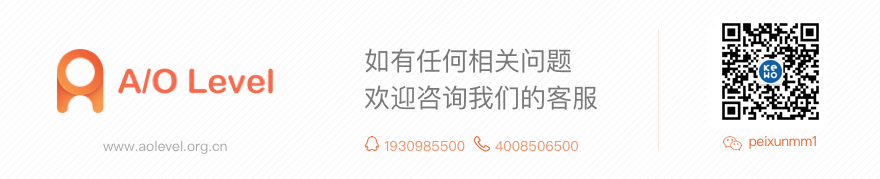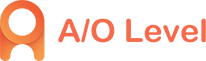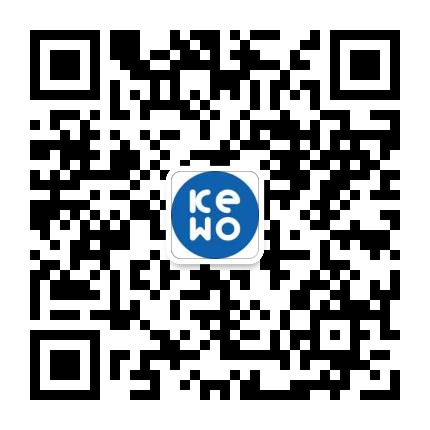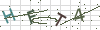• 4008506500
• 1930985500
• peixunmm1
• Suite 601, Level 6, 109 Pitt Street, Sydney,NSW 2000

# A-Level数学考试备考：二次函数与一元二次方程

2019-12-11 10:10:47 555人已读 分享到

A-Level数学考试一直都很受中国考生的欢迎，所考查的知识点基本上在初三以及高中都学过了，所以总体难度不大，但是大家还是要认真将要考察的知识点过一遍，本文小编就为大家简单介绍一下A-Level数学考试二次函数与一元二次方程的内容，希望能够对大家有所帮助。

二次函数与一元二次方程

特别地，二次函数(以下称函数)y=ax^2+bx+c，当y=0时，二次函数为关于x的一元二次方程(以下称方程)，

即ax^2+bx+c=0

此时，函数图像与x轴有无交点即方程有无实数根。

函数与x轴交点的横坐标即为方程的根。

1.二次函数y=ax^2，y=a(x-h)^2，y=a(x-h)^2+k，y=ax^2+bx+c(各式中，a≠0)的图象形状相同，只是位置不同，它们的顶点坐标及对称轴如下表：

解析式 顶点坐标对 称 轴

y=ax^2(0，0) x=0

y=a(x-h)^2(h，0) x=h

y=a(x-h)^2+k(h，k) x=h

y=ax^2+bx+c(-b/2a，[4ac-b^2]/4a) x=-b/2a

当h>0时，y=a(x-h)^2的图象可由抛物线y=ax^2向右平行移动h个单位得到，

当h<0时，则向左平行移动|h|个单位得到.

当h>0,k>0时，将抛物线y=ax^2向右平行移动h个单位，再向上移动k个单位，就可以得到y=a(x-h)^2+k的图象;

当h>0,k<0时，将抛物线y=ax^2向右平行移动h个单位，再向下移动|k|个单位可得到y=a(x-h)^2+k的图象;

当h<0,k>0时，将抛物线向左平行移动|h|个单位，再向上移动k个单位可得到y=a(x-h)^2+k的图象;

当h<0,k<0时，将抛物线向左平行移动|h|个单位，再向下移动|k|个单位可得到y=a(x-h)^2+k的图象;

因此，研究抛物线 y=ax^2+bx+c(a≠0)的图象，通过配方，将一般式化为y=a(x-h)^2+k的形式，可确定其顶点坐标、对称轴，抛物线的大体位置就很清楚了.这给画图象提供了方便.

2.抛物线y=ax^2+bx+c(a≠0)的图象：当a>0时，开口向上，当a<0时开口向下，对称轴是直线x=-b/2a，顶点坐标是(-b/2a，[4ac-b^2]/4a).

3.抛物线y=ax^2+bx+c(a≠0)，若a>0，当x ≤ -b/2a时，y随x的增大而减小;当x ≥ -b/2a时，y随x的增大而增大.若a<0，当x ≤ -b/2a时，y随x的增大而增大;当x ≥ -b/2a时，y随x的增大而减小.

4.抛物线y=ax^2+bx+c的图象与坐标轴的交点：

(1)图象与y轴一定相交，交点坐标为(0，c);

(2)当△=b^2-4ac>0，图象与x轴交于两点A(x?，0)和B(x?，0)，其中的x1,x2是一元二次方程ax^2+bx+c=

(a≠0)的两根.这两点间的距离AB=|x?-x?|

当△=0.图象与x轴只有一个交点;

当△<0.图象与x轴没有交点.当a>0时，图象落在x轴的上方，x为任何实数时，都有y>0;当a<0时，图象落在x轴的下方，x为任何实数时，都有y<0.

5.抛物线y=ax^2+bx+c的最值：如果a>0(a<0)，则当x= -b/2a时，y最小(大)值=(4ac-b^2)/4a.

顶点的横坐标，是取得最值时的自变量值，顶点的纵坐标，是最值的取值.

6.用待定系数法求二次函数的解析式

(1)当题给条件为已知图象经过三个已知点或已知x、y的三对对应值时，可设解析式为一般形式：

y=ax^2+bx+c(a≠0).

(2)当题给条件为已知图象的顶点坐标或对称轴时，可设解析式为顶点式：y=a(x-h)^2+k(a≠0).

(3)当题给条件为已知图象与x轴的两个交点坐标时，可设解析式为两根式：y=a(x-x?)(x-x?)(a≠0).

7.二次函数知识很容易与其它知识综合应用，而形成较为复杂的综合题目。因此，以二次函数知识为主的综合性题目是中考的热点考题，往往以大题形式出现.

以上就是本文关于A-Level数学考试二次函数与一元二次方程的内容介绍了，希望能够对大家有所帮助，如果大家还想了解更多关于alevel真题答案查询、alevel真题试卷等方面的内容，可以咨询在线客服，客服会为您解答~• ### O-level物理考试​电磁学知识点介绍4008506500